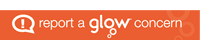# Numeracy and Mathematics

Mathematics equips us with many of the skills required for life, learning and work. Children develop the skills needed to interpret and analyse information, simplify and solve problems, assess risk and make informed decisions

A key feature of the mathematics framework is the development of algebraic thinking from an early stage. Research shows that the earlier algebraic thinking is introduced, the deeper the mathematical understanding will be and the greater the confidence in using mathematics.

Children will develop strategies in order to solve mathematical problems and learn concepts, facts and techniques. The content of the mathematics framework includes Information Handling, Number, Money and Measurement and Shape Position and Movement.

Children are taught basic numeracy skills i.e. addition, subtraction, multiplication and division, as well as developing problem-solving and practical skills and knowledge.  In Robslee Primary, an emphasis is placed upon the development of mental maths strategies and oral and interactive activities. Mental maths has a significant role to play in developing mental agility and, to this end, some time is spent on this area of maths in each class on a daily basis.  Purposeful play in maths helps provide relevance for our children.